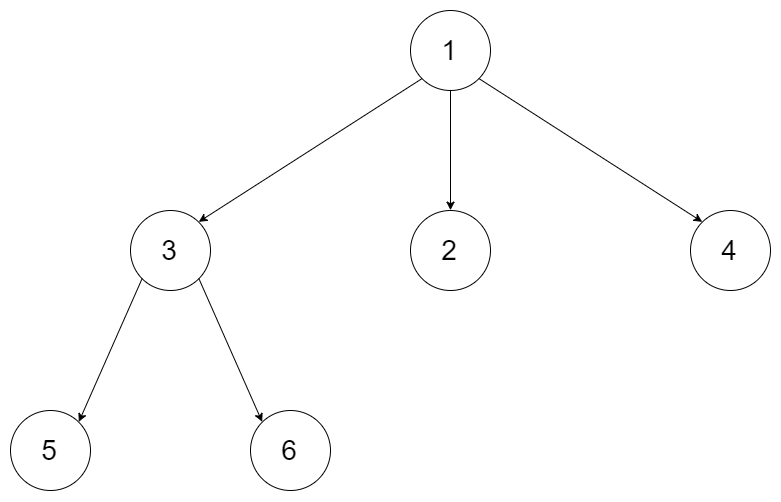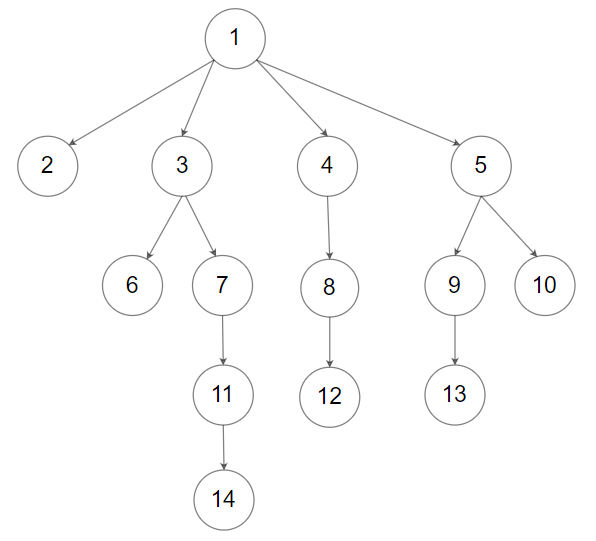# GeetCode Hub

Given a n-ary tree, find its maximum depth.

The maximum depth is the number of nodes along the longest path from the root node down to the farthest leaf node.

Nary-Tree input serialization is represented in their level order traversal, each group of children is separated by the null value (See examples).

Example 1:```Input: root = [1,null,3,2,4,null,5,6]
Output: 3
```

Example 2:```Input: root = [1,null,2,3,4,5,null,null,6,7,null,8,null,9,10,null,null,11,null,12,null,13,null,null,14]
Output: 5
```

Constraints:

• The depth of the n-ary tree is less than or equal to `1000`.
• The total number of nodes is between `[0, 104]`.

/* // Definition for a Node. class Node { public int val; public List<Node> children; public Node() {} public Node(int _val) { val = _val; } public Node(int _val, List<Node> _children) { val = _val; children = _children; } }; */ class Solution { public int maxDepth(Node root) { } }